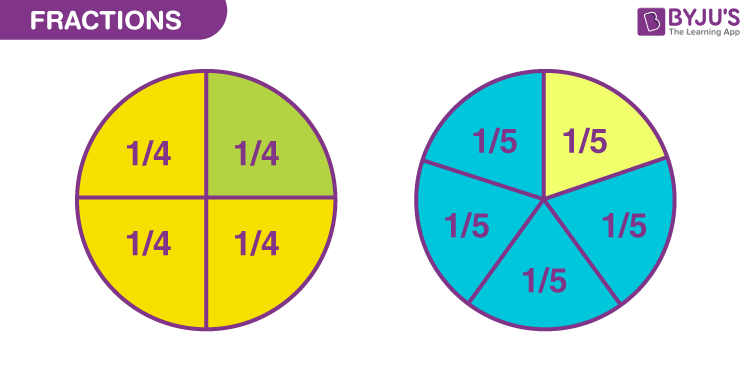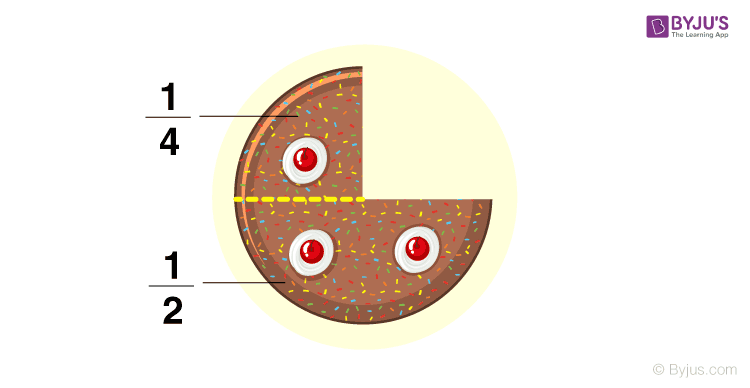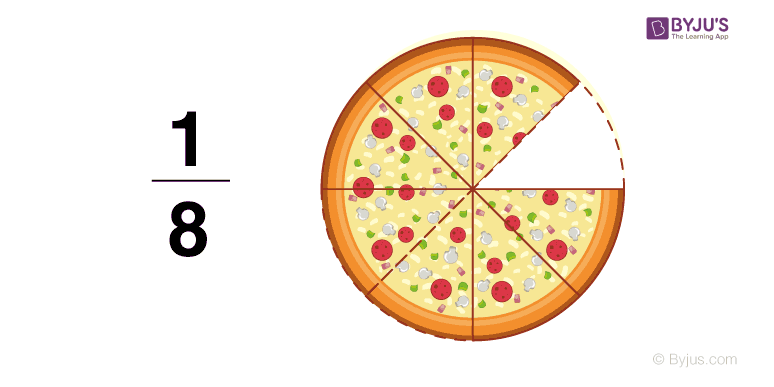# Fraction

Fraction is defined as a part of the whole thing and is also termed as a portion or section of any quantity.

Fraction is written as Numerator / Denominator.

A fraction has two parts, namely numerator and denominator.

The number on the top is called the numerator, and the number on the bottom is called the denominator.

Total selected equal parts from a unit or group represent the numerator. Total equal parts of a unit (whole) or group represent the denominator.

1/2, 2/4, 4/6, 6/12 are a few examples of fractions.

In ½, 1 is the numerator, and 2 is the denominator.• If any object is divided into two equal portions, each portion represents 1/2, which is called half.
• If any object is divided into three equal parts, and out of them, one portion is coloured, then the fraction for the coloured portion will be written as 1/3, called one-third.
• If any object is divided into four equal parts, and out of them, one portion is coloured, the fraction for the coloured portion will be written as 1/4, and it is called one-fourth.
• If any object is divided into four equal parts, and out of them, three portions are coloured, then the fraction for the coloured portion will be written as 3/4, called three-fourth.

## Examples of FractionsStay tuned to BYJU’S for more information on NIOS, syllabus, notes, along with its important questions and solutions.